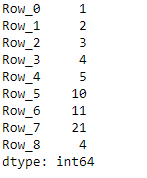• Last Updated : 16 Nov, 2018

Python is a great language for doing data analysis, primarily because of the fantastic ecosystem of data-centric python packages. Pandas is one of those packages and makes importing and analyzing data much easier.

`Dataframe.add_prefix() `function can be used with both series as well as dataframes.

• For Series, the row labels are prefixed.
• For DataFrame, the column labels are prefixed.
```Syntax:  DataFrame.add_prefix(prefix)

Parameters:
prefix : string

Returns: with_prefix: type of caller
```

Example #1: Prefix `col_ `in each columns in the dataframe

 `# importing pandas as pd ` `import` `pandas as pd ` ` `  `# Making data frame from the csv file ` `df ``=` `pd.read_csv(``"nba.csv"``) ` ` `  `# Printing the first 10 rows of the ` `# dataframe for visualization ` `df[:``10``] ``# Using add_prefix() function  ` `# to add 'col_' in each column label ` `df ``=` `df.add_prefix(``'col_'``) ` ` `  `# Print the dataframe ` `df  `

Output:Example #2: Using `add_prefix()` with Series in pandas

`add_prefix()` alters the row index labels in the case of series.

 `# importing pandas as pd ` `import` `pandas as pd ` ` `  `# Creating a Series  ` `df ``=` `pd.Series([``1``, ``2``, ``3``, ``4``, ``5``, ``10``, ``11``, ``21``, ``4``]) ` ` `  `# This will prefix 'Row_' in  ` `# each row of the series ` `df ``=` `df.add_prefix(``'Row_'``) ` ` `  `# Print the Series ` `df `

Output:My Personal Notes arrow_drop_up
Recommended Articles
Page :